# Blades Select Gustafsson in CHL Import Draft

r

Gustafsson, a 1991-born player from Malmo, Sweden, split last season between the Malmo Redhawks senior team and the Malmo Redhawks under-20 junior team. The 5’10, 174 pounder was also a member of Team Sweden at the Under-18 Four Nations Tournament in November at Lake Placid, New York and the Under-18 Five Nations Tournament in February at Nykoping, Sweden.

r

r

r

The selection of Gustafsson, along with returnee 1990-born Finnish defenseman Jyri Niemi, gives the Blades two import players for the upcoming season. Slovak Center Milan Kytnar will remain on the Blades protected list after signing with the NHL’s Edmonton Oilers. The overager is expected to turn pro this fall.

r

r

r

“Hampus is a kid who is a hard working player that plays a strong two-way game,” says Blades General Manager and Head Coach Lorne Molleken. “We look forward to seeing him at training camp in the fall.”

r

r

r

r

Hampus Gustafsson
r5’10, 174 lbs.
rApril 22, 1991
rMalmo, Sweden
rShoots: Left

r

r

r
r
r
r

r

r

r

r

r

r

r

r

r

r

r

r

r

r

r

r

r

r

r

r

r

r

r

r

r

r

r

r

r

r

r

r

r

r

r

r

r

r

r

r

r

r

r

r

r

r

r

r

r

r

r

r

r

r

r

r

r

r

r

r

r

r

r

r

r

r

r

r

r

r

r

r

r

r

r

r

r

r

r

r

r

r

r

r

r

r

r

r

r

r

r

r

r

r

r

r

r

r

r

r

r

r

r

r

r

r

r

r

r

r

r

r

r

r

r

r

r

r

r

r

r

r

r

r

r

r

r

r

r

r

 r 2006-07 r r Skåne r r TV-Pucken r r 8 r r 1 r r 1 r r 2 r r 6 r r   r r Malmo J18 r r J18 Elit r r 2 r r 0 r r 0 r r 0 r r 0 r r   r r Team Sweden U16 (Total) r r Int r r 8 r r 5 r r 3 r r 8 r r 10 r r 2007-08 r r Skåne r r TV-Pucken r r 8 r r 3 r r 6 r r 9 r r 24 r r   r r Malmo J18 r r J18 Elit r r 16 r r 6 r r 14 r r 20 r r 58 r r   r r Malmo J18 r r J18 Allsvenskan r r 13 r r 3 r r 10 r r 13 r r 30 r r   r r Malmo J20 r r SuperElit r r 10 r r 0 r r 1 r r 1 r r 14 r r   r r Team Sweden U17 (Total) r r Int r r 3 r r 0 r r 1 r r 1 r r 2 r r 2008-09 r r Malmo J18 r r J18 Elit r r 1 r r 0 r r 4 r r 4 r r 2 r r   r r Malmo J18 r r J18 Allsvenskan r r 2 r r 2 r r 1 r r 3 r r 2 r r   r r Malmo J20 r r SuperElit r r 31 r r 15 r r 17 r r 32 r r 53 r r   r r Malmo r r Allsvenskan r r 20 r r 1 r r 0 r r 1 r r 6 r r   r r Team Sweden U18 r r Int r r 10 r r 4 r r 3 r r 7 r r 4 r

r

More News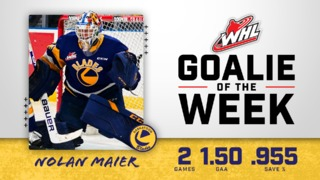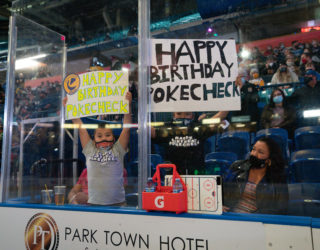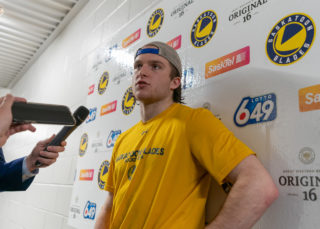3:08
POST GAME | Forward Brandon Lisowsky
12 hours ago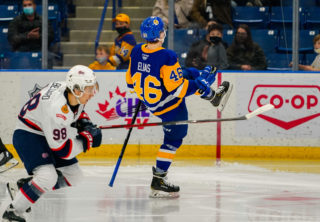2:17
HIGHLIGHTS | Pats (1) Blades (4)
24 hours ago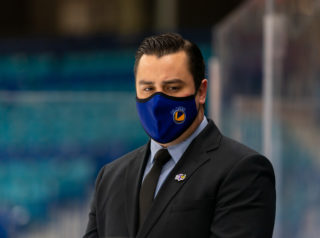3:47
POST GAME | Head coach Brennan Sonne
24 hours ago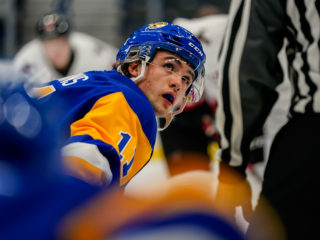2:41
POST GAME | Forward Tristen Robins
24 hours ago